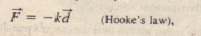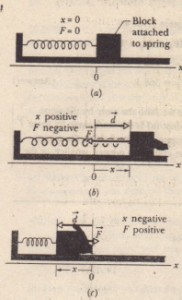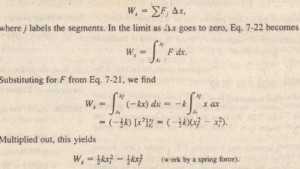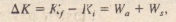# Work Done by a Spring Force

Work Done by a Spring Force

We next want to examine the work done on a particle like object by a  articular  variable force-namely, a spring force, the force from a spring. Many forces in nature have the same mathematical form as the spring force. Thus, by examining this one force, you can gain an understanding of many others.

The Spring Force

Shows a spring in its relaxed state-that is, neither compressed nor extended. One end is fixed, and a particle-like object, say, a block, is attached to the other, free end. If we stretch the spring by pulling the block to the right as the spring pulls on the block toward the left. (Because a spring’s force acts to restore the relaxed state, it is sometimes said to be a restoring force.) If we compress the spring by pushing the block to the left as he spring now pushes on the block toward the right.An x axis has been placed parallel to the length of a spring, with
the origin (x = 0) at the position of the free end when the spring is in its relaxed state. For this common arrangement.

Note that a spring force is a variable force because its magnitude and direction depend on the position x of the free end; F can be symbolized as F(x). Also note  that Hooke’s law is a linear relationship between F and x.(a) A spring in its relaxedstate. The origin of an x axis has beenplaced at the end of the spring that isattached to a block. (b) The block isdisplaced by d, and the spring isstretched by a positive amount x. Notethe restoring force if exerted by thespring. (c) The spring is compressedby a negative amount x. Again, notethe restoring force.

The Work Done by a Spring Force

To find an expression for the work done by the spring force as the block in  moves, let us make two simplifying assumptions about the spring. (I) It is mass less; that is, its mass is negligible compared to the block’s mass. (2) It is an ideal spring; that is, it obeys Hooke’s law exactly. Let us also assume that the contact between the block and the floor is friction less and that the block is particle like.

With the force now constant in each segment, we can find the work done within each segment by using. Then the work done in segment segment 2, and soon. The net work  W. done by the spring, from the sum of all these works.This work W. done by the spring force can have a positive or negative value, depending on whether the net transfer from the block as the block moves.

Work  is positive if the block ends up closer to the relaxed position (x = 0) than it was initially. It is negative if the block ends ? farther away from is zero if the block ends up at the same distance.

The Work Done by an Applied Force

Now. suppose that we displace the block along the X axis while continuing 10 apply a force Fa to it. During the displacement, our applied force does work  on the block while the spring force does work , the change in the kinetic energy of the block due to energy transfers.Caution: If the block is not stationary before-and after the displacement. then this statement is not true.

CHECKPOINT 4: For three situations, the initial and final positions. respectively, along
the x axis for the block in each situation is the work done by the spring force on the block positive, negative.